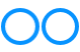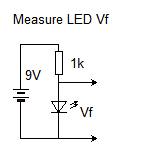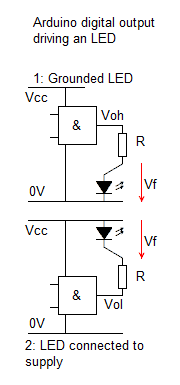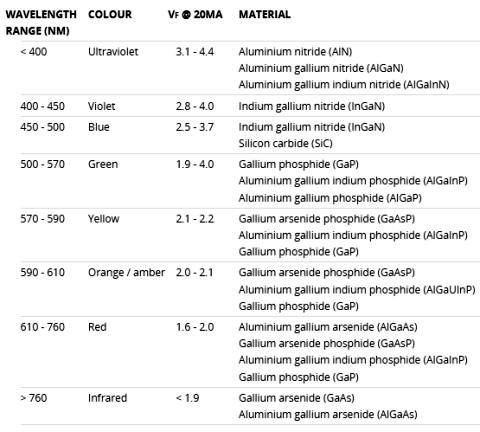# John Errington's Experiments with an Arduino

## Driving LEDs from an Arduino microcontroller

### Easy way to measure VfIf you dont have a data sheet all you need to do to get a useful (not accurate) idea of the Vf is a 9v battery and a 1k resistor. Except for Infra-red, most LEDs have a Vf of about 2-3V so a 9V battery will push 6-7mA through the 1k resistor. Then just use a multimeter (or an arduino) to measure the voltage across the LED.

### How to drive an LED indicator#### I measured the forward voltage of some cheap LED's at a current of 10mA; 3mm and 5mm sizes showed the same values. Here are the results:

NOTE: for use as indicators indoors, red, orange and yellow LEDs AND also blue and white ones are typically acceptable at currents of about 1mA.

While common 3mm and 5mm leds CAN be run at 20mA and more, a maximum of 10mA is generally sufficient, and limits your consumption, especially if you have several inicator lights. (See below for limit of consumption on output ports.)

Green LEDs are MUCH less efficient than red - yellow ones, and need a higher current if their intensities need to match.

The logic gate box in the diagram is just depicting a single arduino digital output pin.

 Colour Vf at 10mA Comment infra-red 1.2 IR 850nm red 2.0 bright amber 2.0 bright green 1.9 very dim blue 2.85 dim white 2.80 bright

#### Table 1 - the "right" resistor value for your project - values for use in daylight:

For use as indicators indoors you can even use resistors up to 10k to minimise power consumption. Try it.

Supply voltage Vcc
LED colour
Current mA
Resistor
3.3
red-green
2.5
470
3.3
red-green
10
120
3.3
blue, white
2.5
180
3.3
blue, white
10
39
5.0
red-green
2.5
1200
5.0
red-green
10
270
5.0
blue, white
2.5
820
5.0
blue, white
10
220

### Further explanation

The table above gives some "rule of thumb" figures. If you want to know how these were calculated , read on.

### LED forward voltage

Here is a table (Table 2) showing the forward voltage for different LEDs in the common 3mm or 5mm package. Its compiled from various manufacturer's data sheets. If you need precise values consult the data sheet for the device you are using.You can see that LEDs in the "warm" end of the spectrum only need low forward voltages, while some types in the "cool" end - green onwards - may need over 3.3V so you could not drive these directly from a 3.3V model arduino.

We also need to bear in mind the electrical characteristics of the arduino chip; each output is capable of sourcing or sinking 40mA; however "the maximum current for each I/O port should not exceed 100mA".

(32U4 data sheet page 379)

If we connected 8 LEDs drawing 20mA each to a single port we could be drawing 8*20mA = 160mA.

### What current do you need?

Assuming you are using the LEDs as indicators, rather then e.g. for a light show, modern LEDs work very well at currents of 2-10mA, and a maximum of 20mA. Higher power LEDs will take more current, but then its better to use a ready-made LED driver chip.

### What is the supply voltage?

The voltage available to drive your LED from a digital output is the difference between the voltage on the digital output pin and the forward voltage of the LED. The data sheet for the ATMega 32U4 gives the following MAXIMUM values (29.0 -29.2) for Vcc = 3.0 or 5.0V. The values I measured for Vol were typically much lower - around 0.1V

Vcc
Iol or Ioh
Vol
Voh
5.0
10mA
0.7
4.2
3.0
5mA
0.5
2.3
3.3 *
5mA
0.55
2.6

* not on data sheet - extrapolated.

### And finally - the calculation

Suppose we wish to drive a red LED with a series resistor, connected between a digital output pin and ground on a 5V Arduino. We choose a current of 10mA.

The voltage available to drive current through the LED is Vcc -Voh = 4.2V (- 0V) and the LED forward voltage (from the data sheet) is 2.1V at 10mA.

The voltage across the resistor Vr will be 4.2 - 2.1 = 2.1V

By ohms law (V / I = R where V=volts, I=Amps, R=Ohms) we get

R = 2.1 / 10 * 10^-3 = 210 ohms.

The nearest preferred value is 220 ohms.

Reference: Table 2